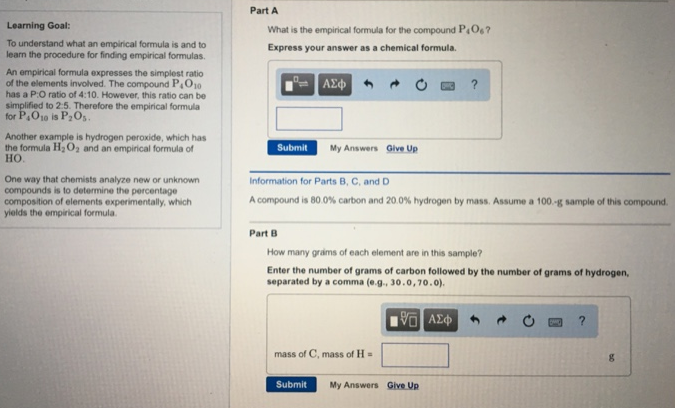# Problem: To understand what an empirical formula is and to team the procedure for finding empirical formulas. An empirical formula expresses the simplest ratio of the elements involved. The compound P4O10 has a P.O ratio of 4:10. However this ratio can be simplified to 2:5. Therefore the empirical formula for P4O10 is P2O5. Another example is hydrogen peroxide, which has the formula H2O2 and an empirical formula of HO. One way that oho mists analyze new or unknown compounds it to determine the percentage composition of elements experimentally, which yields the empirical formula.Part AWhat is the empirical formula for the compound P4O6? Express your answer as a chemical formula. A compound is 80.0% carbon and 20.0% hydrogen by mass. Assume a 100 -g sample of this compound. Part BHow many grams of each element ore in this sample? Enter the number of grams of carbon followed by the number of grams of hydrogen, separated by a comma (e g , 30.0, 70.0).

###### FREE Expert Solution
82% (212 ratings)###### Problem Details

To understand what an empirical formula is and to team the procedure for finding empirical formulas. An empirical formula expresses the simplest ratio of the elements involved. The compound P4O10 has a P.O ratio of 4:10. However this ratio can be simplified to 2:5. Therefore the empirical formula for P4O10 is P2O5. Another example is hydrogen peroxide, which has the formula H2O2 and an empirical formula of HO. One way that oho mists analyze new or unknown compounds it to determine the percentage composition of elements experimentally, which yields the empirical formula.

Part A

What is the empirical formula for the compound P4O6

A compound is 80.0% carbon and 20.0% hydrogen by mass. Assume a 100 -g sample of this compound.

Part B

How many grams of each element ore in this sample?

Enter the number of grams of carbon followed by the number of grams of hydrogen, separated by a comma (e g , 30.0, 70.0).